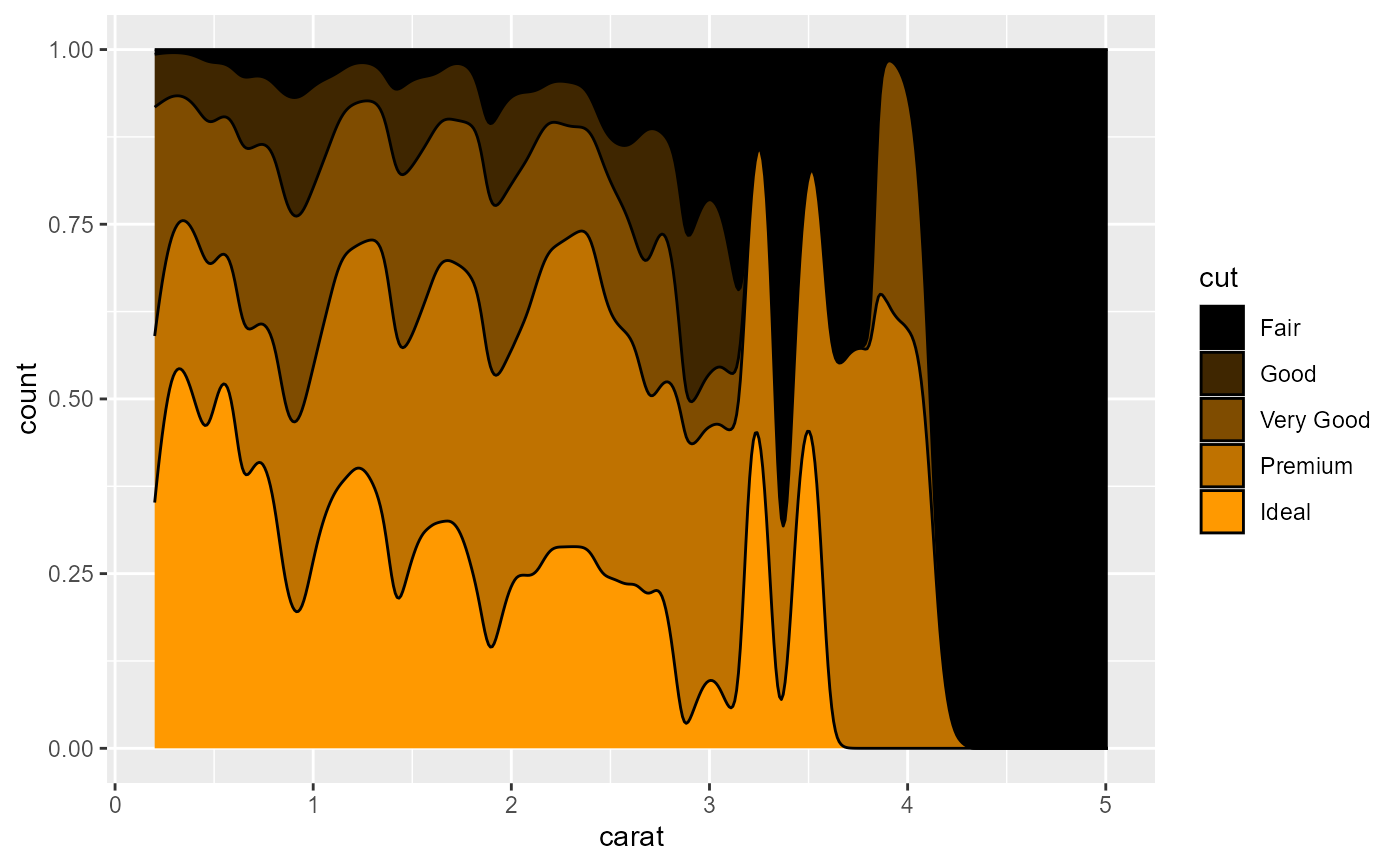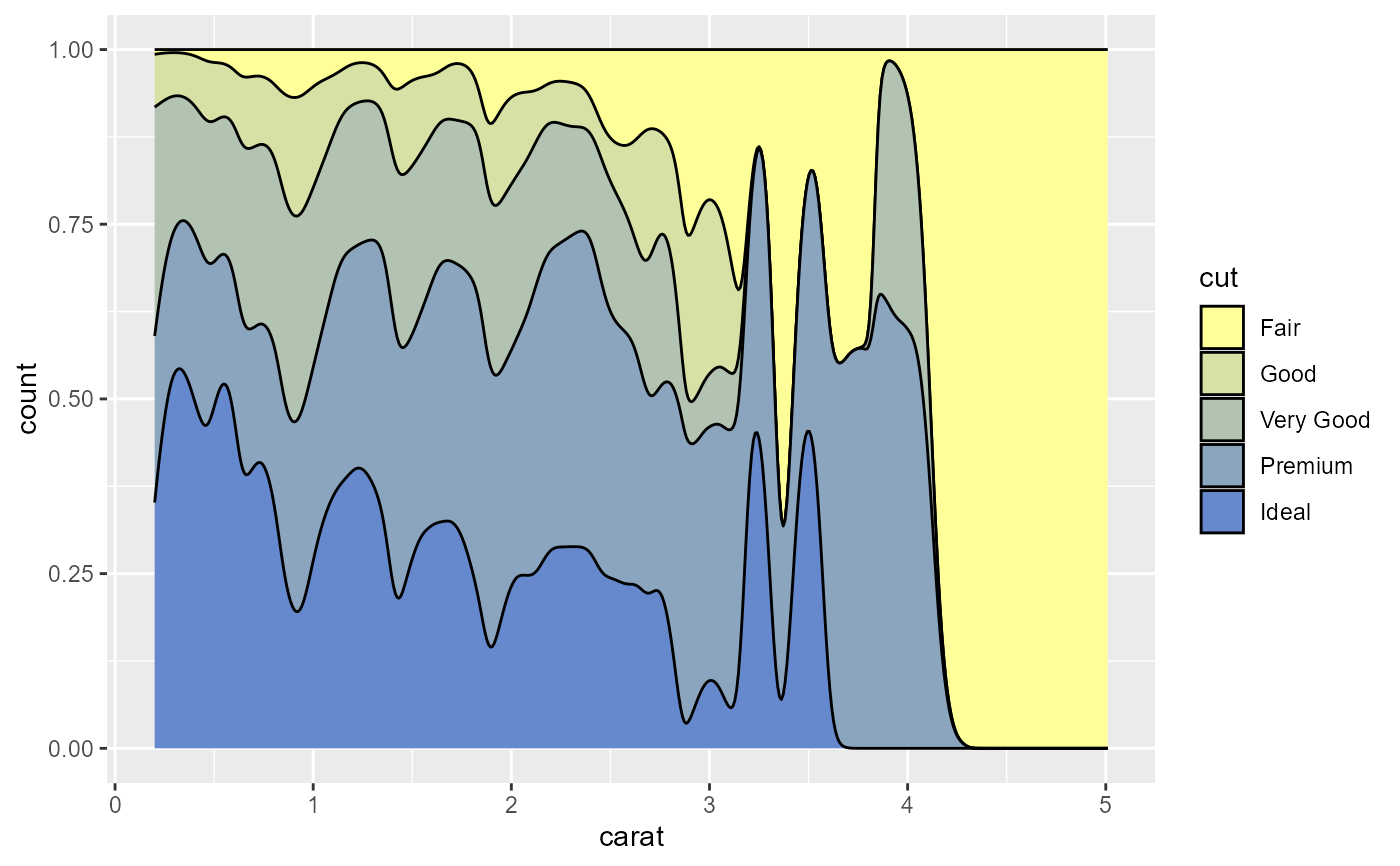Scale functions used with ggplot2.

scale_color_lcars(palette = "2357", discrete = TRUE, reverse = FALSE, ...)

scale_color_lcars1(
color = "atomic-tangerine",
discrete = TRUE,
reverse = FALSE,
dark = FALSE,
...
)

scale_color_lcars2(
low = "atomic-tangerine",
high = "near-blue",
discrete = TRUE,
reverse = FALSE,
dark = FALSE,
divergent = FALSE,
...
)

scale_fill_lcars(palette = "2357", discrete = TRUE, reverse = FALSE, ...)

scale_fill_lcars1(
color = "atomic-tangerine",
discrete = TRUE,
reverse = FALSE,
dark = FALSE,
...
)

scale_fill_lcars2(
low = "atomic-tangerine",
high = "near-blue",
discrete = TRUE,
reverse = FALSE,
dark = FALSE,
divergent = FALSE,
...
)

## Arguments

palette character, name of palette in lcars_pals. logical, discrete or continuous palette. logical, reverse color order. additional arguments passed to ggplot2::discrete_scale or ggplot2::scale_*_gradientn, for discrete or continuous palettes, respectively. character, LCARS color name for sequential palette. logical, use black instead of white for the base color in sequential palette or midpoint in divergent palette. character, LCARS color name. character, LCARS color name. logical, use a divergent palette instead of two-color sequential palette.

## Examples

library(ggplot2)
p <- ggplot(diamonds, aes(carat, stat(count), fill = cut)) +
geom_density(position = "fill")
p + scale_fill_lcars("2357")p + scale_fill_lcars1("atomic-tangerine", dark = TRUE)p + scale_fill_lcars2("pale-canary", "danub")